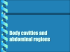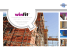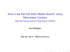# 498 Chapter 13. Quantifying Uncertainty

## Transcription

498 Chapter 13. Quantifying Uncertainty
```498
Chapter 13.
Quantifying Uncertainty
approximate methods for evidence combination that, while giving incorrect answers, require
fewer numbers to give any answer at all.
Rather than taking this route, we need to find some additional assertions about the
domain that will enable us to simplify the expressions. The notion of independence in Sec-
tion 13.4 provides a clue, but needs refining. It would be nice if Toothache and Catch were
independent, but they are not: if the probe catches in the tooth, then it is likely that the tooth
has a cavity and that the cavity causes a toothache. These variables are independent, however, given the presence or the absence of a cavity. Each is directly caused by the cavity, but
neither has a direct effect on the other: toothache depends on the state of the nerves in the
tooth, whereas the probe's accuracy depends on the dentist's skill, to which the toothache is
irrelevant. 5 Mathematically, this property is written as
CONDITIONAL
INDEPENDENCE
P(toothache A catch I Cavity) = P(toothache I Cavity)P(catch I Cavity) . (13.17)
This equation expresses the conditional independence of toothache and catch given Cavity.
We can plug it into Equation (13_16) to obtain the probability of a cavity:
P( Cavity I toothache A catch)
= orP(tooth.ache Cavity)P(catch Cavity)P(Cavity) .
(13.18)
Now the information requirements are the same as for inference, using each piece of evidence separately: the prior probability P (Cavity) for the query variable and the conditional
probability of each effect, given its cause.
The general definition of conditional independence of two variables X and Y, given a
third variable 7, is
3
P(X, Y Z) = P(X Z)1 (Y I Z) .
In the dentist domain, for example, it seems reasonable to assert conditional independence of
the variables Toothache and Catch, given Cavity:
P(Toothache Catch I Cavity) = P(Toothache Cavity)P(Catch I Cavity) . (13.19)
Notice that this assertion is somewhat stronger than Equation (13.17), which asserts independence only for specific values of Toothache and Catch. As with absolute independence in
Equation (13A1), the equivalent forms
,
P(X I Y, 2) =P( X Z)
and P(Y I X, Z) =P(Y I Z)
can also be used (see Exercise 13.17). Section 13.4 showed that absolute independence assertions allow a decomposition of the full joint distribution into much smaller pieces. It turns
out that the same is true for conditional independence assertions. For example, given the
assertion in Equation (13.19), we can derive a decomposition as follows:
P(Toothache, Catch, Cavity)
= P( Toothache, Catch I Carity)P( Cavity)
(product rule)
= P( Toothache Cavity)P( Catch I Cavity)P( Cavity) (using 13.19).
(The reader can easily check that this equation does in fact hold in Figure 13.3.) In this way.
the original large table is decomposed into three smaller tables. The original table has seven
We assume that the patient and dentist are distinct individuals.
Section 13.6.
rfrSEPARATION
The Wumpus World Revisited
499
independent numbers (2 3 = 8 entries in the table, but they must sum to 1, so 7 are independent). The smaller tables contain five independent numbers (for a conditional probability
distributions such as P(TIC there are two rows of two numbers, and each row sums to 1, so
that's two independent numbers: for a prior distribution like P(C) there is only one independent number). Going from seven to five might not seem like a major triumph, but the point
is that, for ra symptoms that are all conditionally independent given Cavity, the size of the
representation grows as 0(72) instead of 0(2n). That means that conditional independence
assertions can allow probabilistic systems to stale up; Inoreoyer, they are much more commonly available than absolute independence assertions. Conceptually, Cavity separates
Toothache and Catch because it is a direct cause of both of them. The decomposition of
large probabilistic domains into weakly connected subsets through conditional independence
is one of the most important developments in the recent history of Al.
The dentistry example illustrates a commonly UCCILIT1lig pattern in which a single cause
directly influences a number of effects, all of which are conditionally independent, given the
cause. The full joint distribution can be written as
P (Cause, Effect
PN YE BAYES
Effect,,) — P( Cause)llP(Effect,1 Cause) .
Such a probability distribution is called a naive Bayes model—"naive" because it is often
used (as a simplifying assumption) in cases where the "effect" variables are not actually
conditionally independent given the cause variable. (The naive Bayes model is sometimes
called a Bayesian classifier, a somewhat careless usage that has prompted true Bayesians
to call it the idiot Bayes model.) In practice, naive Bayes systems can work surprisingly
well, even when the conditional independence assumption is not true. Chapter 20 describes
methods for learning naive Bayes distributions from observations.
13.6 THE WUMPUS WORLD REVISITED
We can combine of the ideas in this chapter to solve probabilistic reasoning problems in the
wumpus world. (See Chapter 7 for a complete description of the wumpus world.) Uncertainty
arises in the wumpus world because the agent's sensors give only partial information about
the world. For example, Figure 13.5 shows a situation in which each of the three reachable
squares—[1,3], [2,2], and [3,1]—might contain a pit. Pure logical inference can conclude
nothing about which square is most likely to be safe, so a logical agent might have to choose
randomly. We will see that a probabilistic agent can do much better than the logical agent.
Our aim is to calculate the probability that each of the three squares contains a pit. (For
this example we ignore the wumpus and the gold.) The relevant properties of the wumpus
world are that (1) a pit causes breezes in all neighboring squares, and (2) each square other
than [1,1] contains a pit with probability 0.2. The first step is to identify the set of random
variables we need:
•
As in the propositional logic case, we want one Boolean variable Pij for each square,
which is true iff square [i, j] actually contains a pit.
```

### CSE 5522 Homework 1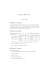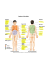### Document 6591906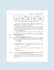### Chap1- anatomical terminology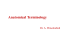### Page 1 HUMAN BODY f Jaim., »om w mnt: #i `/ âïsfemz of *hc body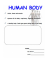### Project sheet - Denby Dale - PASS-NET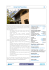### LAB 1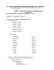### From: Chris Arden [mailto:[email protected]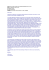### Body cavities and abdominal regions